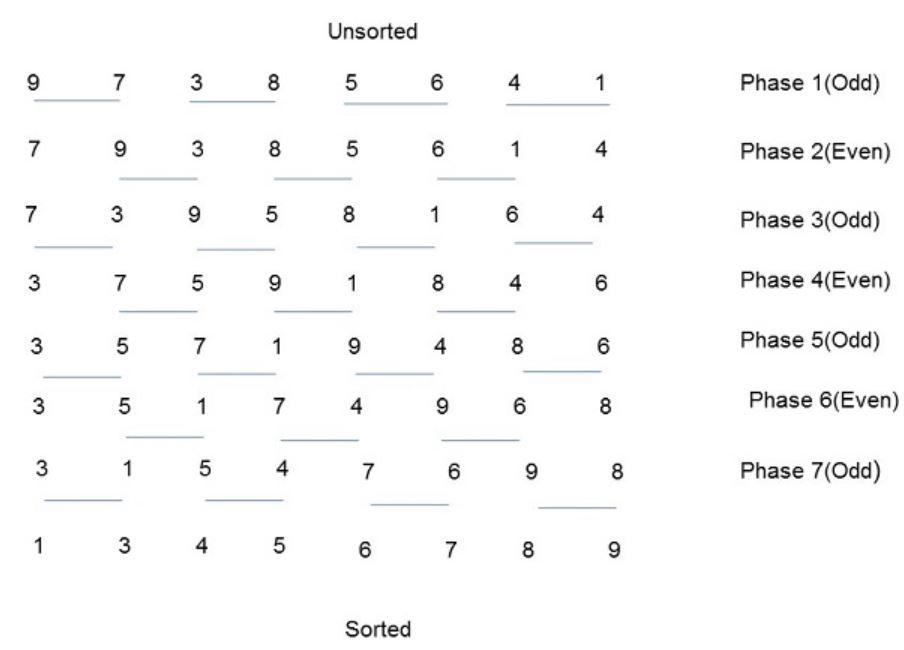×

Search anything:

# Odd Even Sort / Brick Sort

#### Algorithms Sorting Algorithms bubble sort odd even sortGet this book -> Problems on Array: For Interviews and Competitive Programming

Reading time: 20 minutes | Coding time: 5 minutes

Sorting is a process of arranging elements in a group in a particular order, i.e., ascending order, descending order, alphabetic order, etc.
Sorting a list of elements is a very common operation. A sequential sorting algorithm may not be efficient enough when we have to sort a huge volume of data. Therefore, parallel algorithms are used in sorting.

Parallel Algorithm : A parallel algorithm is an algorithm that can execute several instructions simultaneously on different processing devices and then combine all the individual outputs to produce the final result.

Odd Even Sort uses parallel algorithm which is based on bubble sort technique.
It is also known as Brick Sort.

### Algorithm

1. It compares two adjacent numbers and switches them, if the first number is greater than the second number to get an ascending order list.
2. The opposite case applies for a descending order series.
3. Odd-Even transposition sort operates in two phases − odd phase and even phase.
4. In both the phases, processes exchange numbers with their adjacent number in the right.

### Pseudo Code

``````procedure ODD-EVEN_PAR (n)

begin
id := process's label

for i := 1 to n do
begin

if i is odd and id is odd then
compare-exchange_min(id + 1);
else
compare-exchange_max(id - 1);

if i is even and id is even then
compare-exchange_min(id + 1);
else
compare-exchange_max(id - 1);

end for

end ODD-EVEN_PAR
``````

### Example``````Unsorted : 9 7 3 8 5 6 4 1

Phase 1 : 9 > 7 => Swap , 4 > 1 => Swap
Phase 2 : 9 > 3 => Swap , 8 > 5 => Swap , 6 > 1 => Swap
Phase 3 : 7 > 3 => Swap , 9 > 5 => Swap , 8 > 1 => Swap , 6 > 4 => Swap
Phase 4 : 7 > 5 => Swap , 9 > 1 => Swap , 8 > 4 => Swap
Phase 5 : 7 > 1 => Swap , 9 > 4 => Swap , 8 > 6 => Swap
Phase 6 : 5 > 1 => Swap , 7 > 4 => Swap , 9 > 6 => Swap
Phase 7 : 3 > 1 => Swap , 5 > 4 => Swap , 7 > 6 => Swap , 9 > 8 => Swap

Sorted : 1 3 4 5 6 7 8 9
``````

• C++
• Python

### C++

``````
// A C++ Program to implement Odd-Even / Brick Sort
#include<bits/stdc++.h>
using namespace std;
// A function to sort the algorithm using Odd Even sort
void oddEvenSort(int arr[], int n)
{
bool isSorted = false; // Initially array is unsorted
while (!isSorted)
{
isSorted = true;
// Perform Bubble sort on odd indexed element
for (int i=1; i<=n-2; i=i+2)
{
if (arr[i] > arr[i+1])
{
swap(arr[i], arr[i+1]);
isSorted = false;
}
}
// Perform Bubble sort on even indexed element
for (int i=0; i<=n-2; i=i+2)
{
if (arr[i] > arr[i+1])
{
swap(arr[i], arr[i+1]);
isSorted = false;
}
}
}
return;
}
// A utility function ot print an array of size n
void printArray(int arr[], int n)
{
for (int i=0; i < n; i++)
cout << arr[i] << " ";
cout << "\n";
}
// Driver program to test above functions.
int main()
{
int arr[] = {9,7,3,8,5,6,4,1};
int n = sizeof(arr)/sizeof(arr);
oddEvenSort(arr, n);
printArray(arr, n);
return (0);
}
``````

### Python

``````
# Python Program to implement
# Odd-Even / Brick Sort
def oddEvenSort(arr, n):
# Initially array is unsorted
isSorted = 0
while isSorted == 0:
isSorted = 1
temp = 0
for i in range(1, n-1, 2):
if arr[i] > arr[i+1]:
arr[i], arr[i+1] = arr[i+1], arr[i]
isSorted = 0
for i in range(0, n-1, 2):
if arr[i] > arr[i+1]:
arr[i], arr[i+1] = arr[i+1], arr[i]
isSorted = 0
return
arr = [9,7,3,8,5,6,4,1]
n = len(arr)
oddEvenSort(arr, n);
for i in range(0, n):
print(arr[i], end = ' ')
``````

### Complexity

• Worst case time complexity: `Θ(n*n)`
• Average case time complexity: `Θ(n*n)`
• Best case time complexity: `Θ(n)`
• Space complexity: `Θ(1)`

### Question

#### Is this a Stable Sort?

Yes
No
A sorting algorithm is said to be stable if two objects with equal keys appear in the same order in sorted output as they appear in the input unsorted array. Some sorting algorithms are stable by nature like Insertion sort, Merge Sort, Bubble Sort, etc. Since a type of bubble sort it is also stable.#### Harshita Sahai

Maintainer at OpenGenus | Previously Software Developer, Intern at OpenGenus (June to August 2019) | B.Tech in Information Technology from Guru Gobind Singh Indraprastha University (2017 to 2021)

Odd Even Sort / Brick Sort